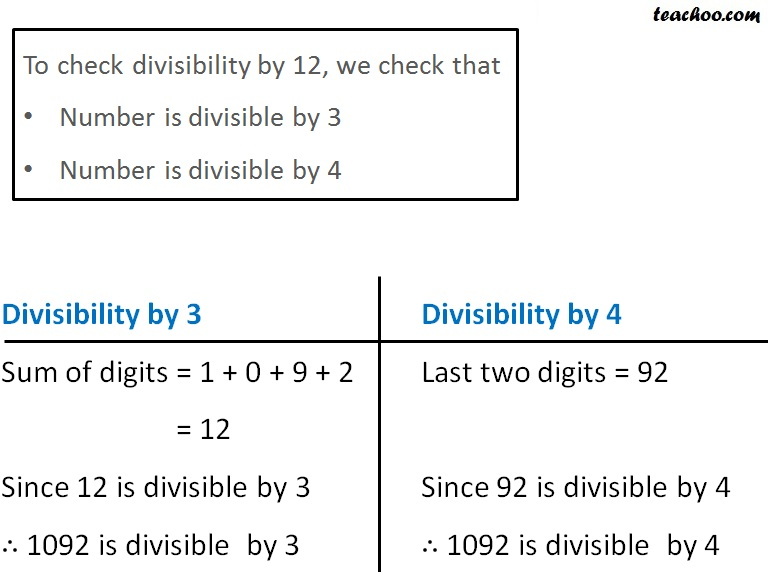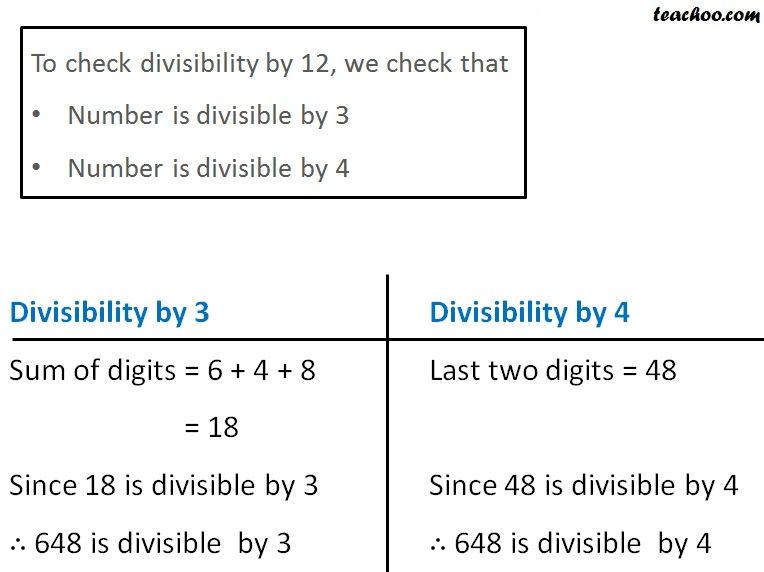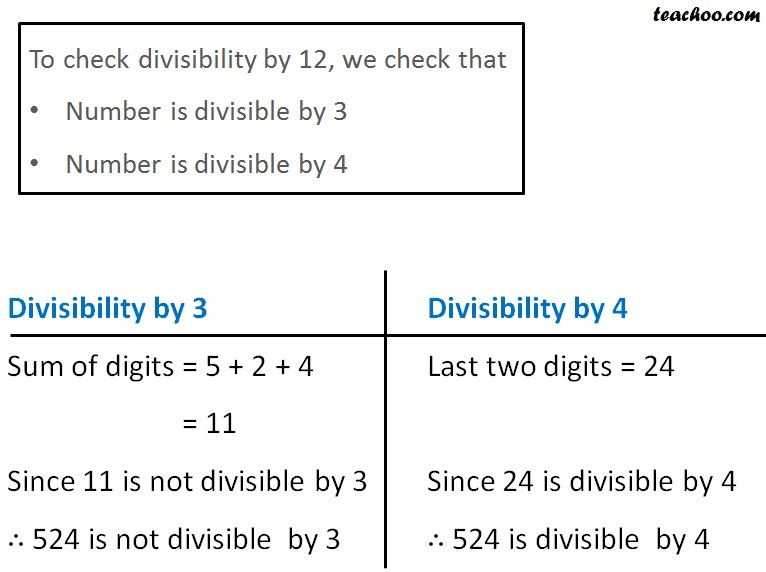Divisibility Tests - All

Chapter 3 Class 6 Playing with Numbers
Concept wise

A number is divisible by 12 if

• It is divisible by 3
• It is divisible by 4

Let’s take some examples

## Is 1092 is divisible by 12?Since 1092 is divisible by both 3 & 4

It is divisible by 12

## Is 648 is divisible by 12?Since 648 is divisible by both 3 & 4

It is divisible by 12

## Is 524 is divisible by 12?Since 524 is not divisible by 3

It is not divisible by 12

Learn in your speed, with individual attention - Teachoo Maths 1-on-1 Class

### Transcript

To check divisibility by 12, we check that Number is divisible by 3 Number is divisible by 4 Divisibility by 3 Sum of digits = 1 + 0 + 9 + 2 = 12 Since 12 is divisible by 3 ∴ 1092 is divisible by 3 Divisibility by 4 Last two digits = 92 Since 92 is divisible by 4 ∴ 1092 is divisible by 4 To check divisibility by 12, we check that Number is divisible by 3 Number is divisible by 4 Divisibility by 3 Sum of digits = 6 + 4 + 8 = 18 Since 18 is divisible by 3 ∴ 648 is divisible by 3 Divisibility by 4 Last two digits = 48 Since 48 is divisible by 4 ∴ 648 is divisible by 4 To check divisibility by 12, we check that Number is divisible by 3 Number is divisible by 4 Divisibility by 3 Sum of digits = 5 + 2 + 4 = 11 Since 11 is not divisible by 3 ∴ 524 is not divisible by 3 Divisibility by 4 Last two digits = 24 Since 24 is divisible by 4 ∴ 524 is divisible by 4